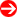#Product Naming Convention - Servers (AMD)Character Representation Options 1st Form Factor • 1 = 1U         • 2 = 2U         • 3 = 3U         • 4 = 4U / Tower         • F1 = FatTwin® 1U node         • F2 = FatTwin® 2U node 2nd HD Tray Type / Chassis • 0 = 3.5"         • 1 = 2.5" 3rd CPU Count • 1 = Single         • 2 = Dual 4th Generation • 3 = 4th Gen. (Socket SP3; Zen) (7001/7002 Family)         • 4 = 5th Gen. (Socket SP3; Zen2/Zen3)                  (7002/7003 Family) 5th & 6th Server Platform • BT = BigTwin®         • C = CloudDC         • G = GPU         • GO = Eight GPU         • GQ = Quad GPU         • GT = GPUTwin (for Twin Family only)         • TP = Twin Server         • TS = Tower         • U = Ultra Server         • S = Socket SP3 (Zen/Zen2/Zen3 [7001/7002/7003]) 7th/8th Interface Type • A = A100 (GPU base)         • D = Dual Node (for GT Family)         • F = Front IO node         • H = Hot Swap         • i = Internal SATA         • M = Short Depth         • N = NVMe         • N10 = 10 NVMe         • N24 = 24 NVMe         • W = WIO         • R = Rear IO node         • T = SATA         • R = Redundant Power (8th) 9th and higher Network • P = 10G SFP+         • T = Ryzen Threadripper PRO 39XX processor                  (for GT Family)         • T = 10G